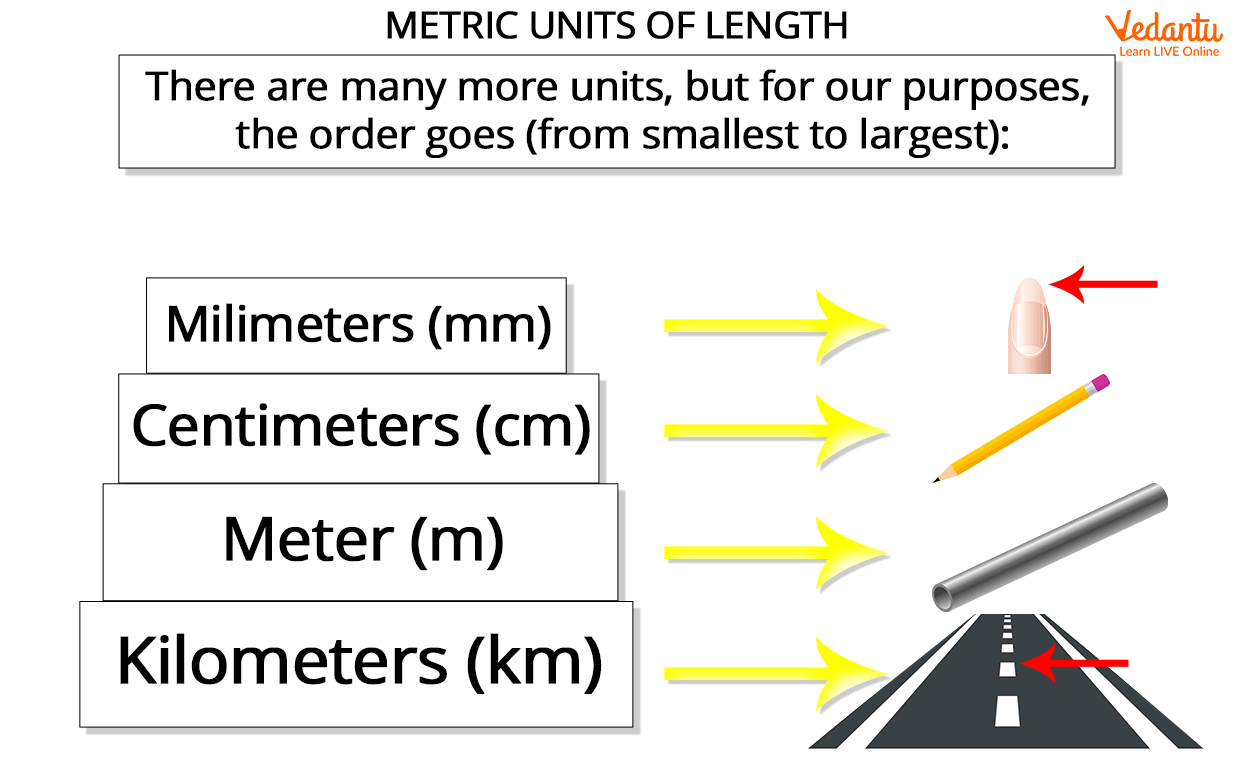Courses
Courses for Kids
Free study material
Free LIVE classes
More

# Kilometre Definition and Its Conversion in MathsLIVE
Join Vedantu’s FREE Mastercalss

## Introduction to Kilometre for Kids

Everyone loves taking long walks or bicycling around a park. But have you ever wondered how the distance that we travel during these walks or bicycling is measured? How can we measure the distance between our home and school? We measure the distance in kilometres. But now we often hear or read on maps that the turn is 10 metres away or the destination metres. What does it mean? Well, the metre is also a term used to quantify the distance.

In this article, we will learn about the metre and kilometre definitions in Maths and their conversion.

## What is the Metric System?

A Metric system is an international system that deals with the use of metric units. These units are used to measure height, weight, distance, or length. The metric system also has units like kilometres and metres that are used to measure the length or the distance of the object. Another example of a metric unit is kilograms, this is used to quantify the mass of a body. Grams is a smaller metric unit that is also used to quantify the mass of an object. The image illustrated below represents the different units that are used to measure length.Metric units used to measure the length

## What is a Kilometre?

Since we have learnt about the metric system, we can now discuss the kilometre definition in Maths. A kilometre is a SI unit that is used to measure distance. The term is abbreviated as km. The prefix kilo is derived from Greek which refers to a thousand. This interesting fact can help kids in understanding the conversion of metric units. Let us look into some examples where kilometres can be used.

• The distance between the school and my home is 2 km.

• The distance between Delhi and Bangalore is 2147 km.

• I go for a 3 km walk every morning.Distance from home to school

## What is a Metre?

A metre is defined as the metric unit that is used to measure smaller distances and lengths. It is abbreviated as (m). The term metre is commonly used to measure lengths that are smaller than 1000 metres. For example, the term is used to measure the length of the table or the length of a Christmas tree. The term is also used to quantify small distances, for instance:

• The turn is 10 metres away.

• There is a basketball court 60 m from the school playground.

## Relation Between Metres and Kilometres

As we have learnt the kilometre definition in Maths and the metre definition as well, let us look into the relationship between them. As km and m are metric units, they can be converted into each other. 1 kilometre is equal to 1000 metres. That means we can express the values of a given distance in both units. The following examples can help students understand the conversion.

Example 1

If a car travels 20 km to reach points A to B, what is the distance travelled by car in metres?

Solution: Since 1 km is equal to 1000 m, to convert the 20 km, we need to multiply 20 by 1000.

20 x1000 = 20000 metres.

Example 2

What is the distance in kilometres if the distance travelled is 3 metre?

Solution: The distance travelled is 3 m.

1 km = 1000 m

1 m = 11000 km

31000=0.003 km

The distance travelled is 0.003 km.

## Conclusion

This was the complete discussion on the kilometre and metre. We have learnt the kilometre definition in Maths as well as its relation with metres. We have also learnt how to convert the length into metres and kilometres. Students can practise more questions to develop a better understanding of the concept.

Last updated date: 16th Sep 2023
Total views: 135.6k
Views today: 1.35k

## FAQs on Kilometre Definition and Its Conversion in Maths

1. What are some other units in which length can be measured?

Miles and feet are some of the units that are used to measure the length. These units are classified under United States Customary Units (USCS). The miles are abbreviated as (mi) whereas the feet is abbreviated as (ft). 1 kilometre is equal to 0.62137 miles and 3,280.84 feet.

2. What is the relation between a kilometre and a centimetre?

A centimetre is also an SI unit that is used to measure the length of an object. Centimetre is abbreviated as (cm). The relation between them is that 1 km is equal to  1,00,000 cm. Further, 1 metre is equal to 100 centimetres.